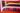# Playing with Go, Functions as a parameter

May 15, 2019 📖 2 min read

There are a few things very terrifying for beginners when we are learning to code. One of them is passing functions as a parameter to other functions.

Functions are first class values in Go: Like other values, functions values have types, and they may be assigned to variables or passed to or returned from functions. A function value may be called like any other funcion. - The Go Programming Language, Alan A. A. Donovan, Brian W. Kernighan

Have you ever wonder why do we pass functions in the first place? If you are an experienced engineer you probably already know the answer. There is a lot of good reads and tutorials about how to pass functions as parameter to other functions, but if you add `why` to the formula, the results are a bit scarce.

The perfect example is a Map() function

``````func Map(n []int, f func(x int) int) []int {
result := make([]int, len(n))
for i, v := range n {
result[i] = f(v)
}
return result
}``````

With the same function we can have different behaviours just changing the callback function! Let’s see another example:

``````package main

import "fmt"

func main() {
var numbers = []int{1, 4, 9, 16}
double := func(n int) int {
return n * 2
}
square := func(n int) int {
return n * n
}
n := Map(numbers, double)
// We just changed the behaviour of Map()
// simply passing another function
s := Map(numbers, square)

fmt.Println(n, s)
}

// Map function
func Map(n []int, f func(x int) int) []int {
result := make([]int, len(n))
for i, v := range n {
result[i] = f(v)
}
return result
}``````

In this example, as we can see, `double` and `square` are both variables with function values of type `func (int) int`, remember our definition at the beginning? The important thing here is that we didn’t modified our Map function. Instead, we added the flexibility to parametrize it’s behaviour thanks to the function callbacks! How awesome is that?

And this is one example why languages that let you treat functions as a first class values are so powerful!

Please, keep in mind that I’m learning! if you find something that can be optimized or better explained let me know! I’d love to hear about it!